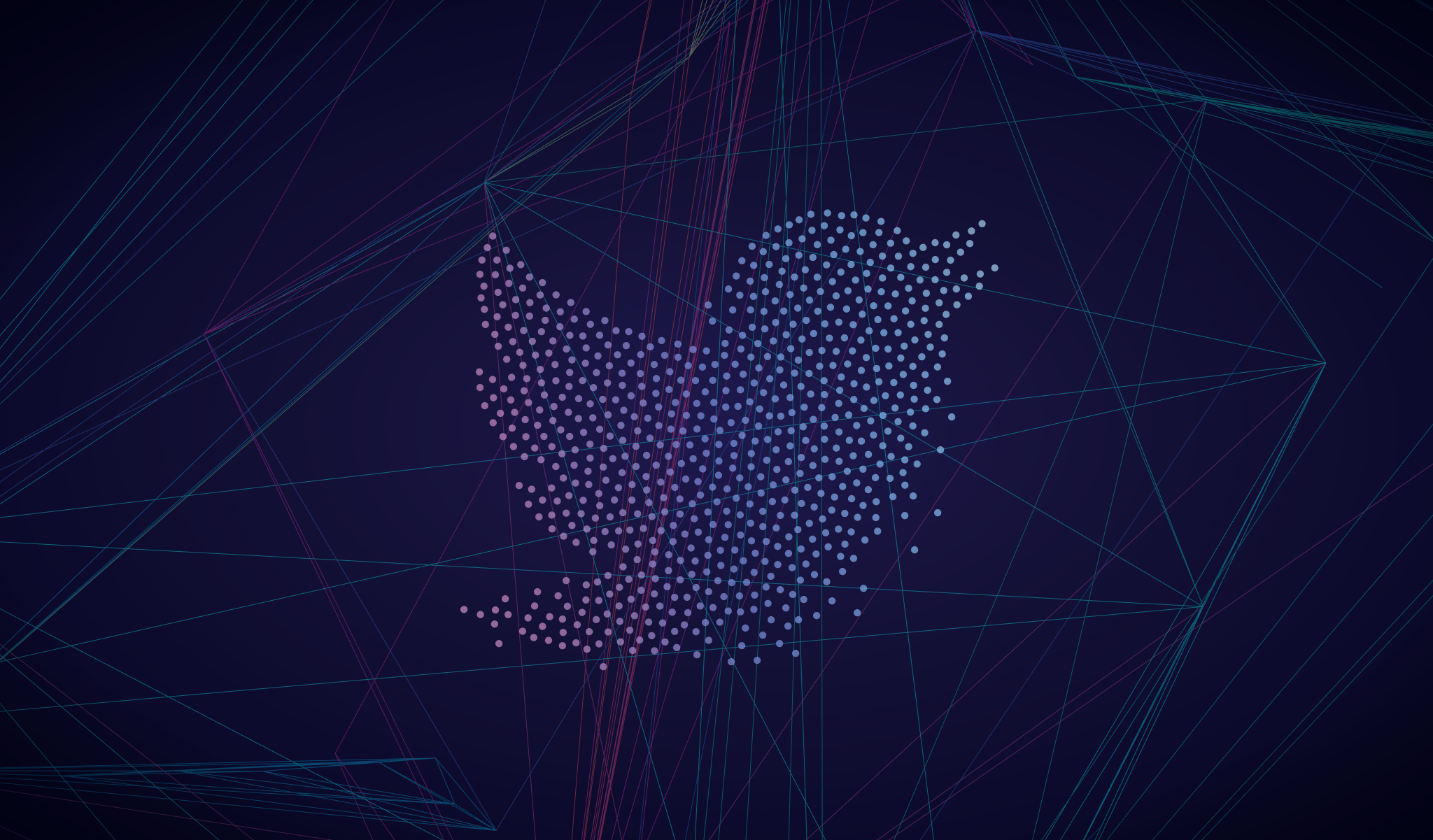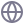+00:00 GMT
• Home
• Events
• Content
• People
• Messages
• Channels
• Help
Articles
June 2, 2022

# How to Build an Autoencoder Using TensorFlow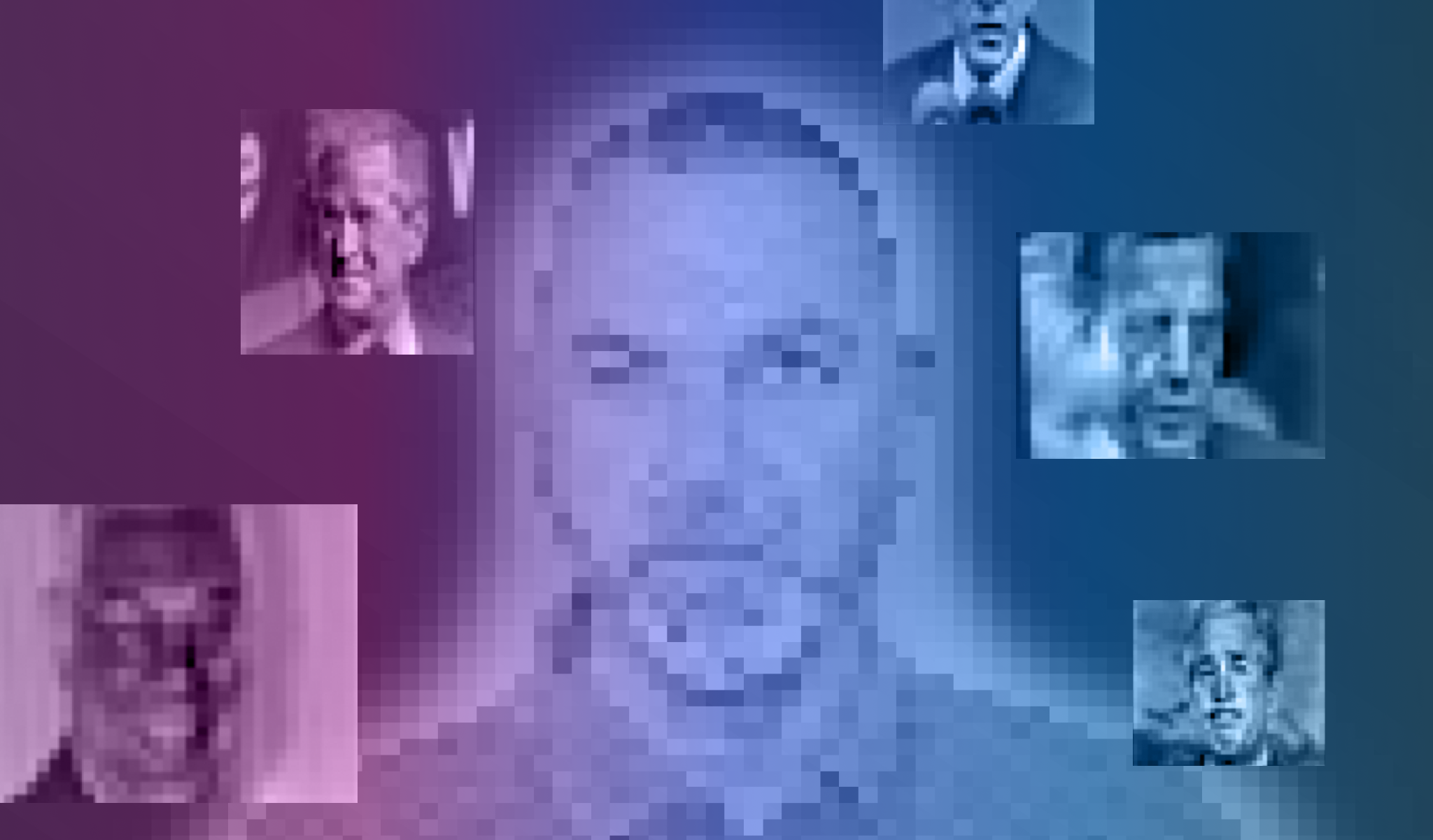# TensorFlow
# Tutorial
# Keras
# Autoencoder

## Here's how to build an autoencoder for image compression, image reconstruction, and supervised learning using the TensorFlow library.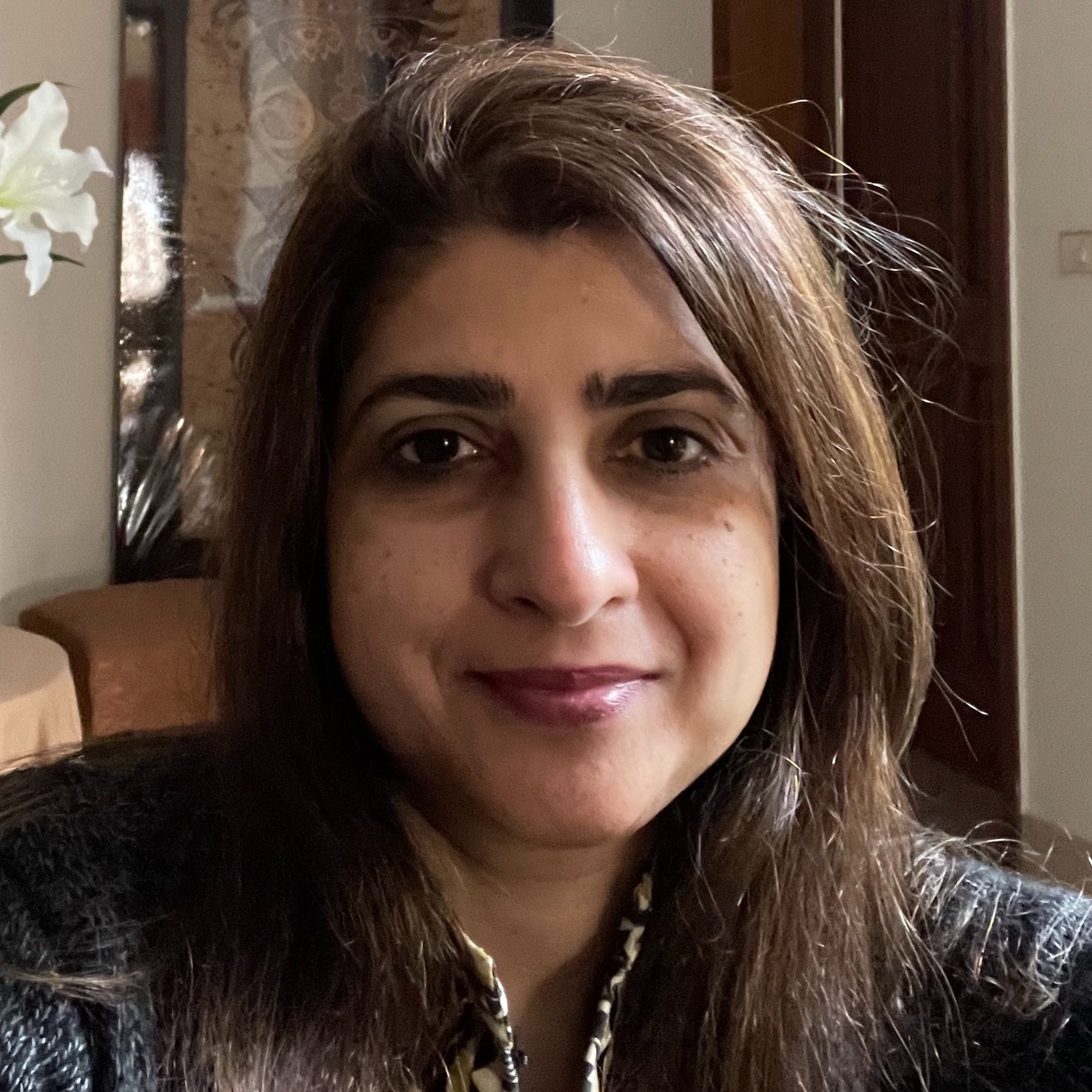Mehreen SaeedIn this article, I'll discuss using TensorFlow for supervised classification tasks, and we’ll work with a dataset of faces to build a simple autoencoder. We’ll use it for reconstructing the original face images and also visualize the latent space and build a supervised classifier from it that performs face recognition. For the implementation part, we’ll use TensorFlow and Keras library to build our model.

An autoencoder has two parts: an encoder and a decoder. The encoder learns a latent representation of the input data, and the decoder is trained to reconstruct the original inputs from the latent representations. The autoencoder has the following applications.

1. It autoencoder approximates the original input points from the latent representations. This makes it useful for data recovery from corrupt inputs.
2. As the autoencoder learns a latent representation of the input data, it can be designed so that the dimensions of this latent space is much smaller than the original input dimensions. Hence, an autoencoder can be used for data compression.
3. Autoencoders find their application for data augmentation. The outputs from the autoencoder represent synthetic data and, hence, can be added to the original training set to increase its size.
4. You can use the latent representation from an autoencoder to learn classification and regression tasks.

Here's how to get started building your own autoencoder. (Note: You can run the code shown in this tutorial in Google Colab or download the Python notebook here. Output shown from the code examples this article will not match the output that you get at your end: The output will vary with each run of the program because of the stochastic (random) nature of the algorithms involved.)

## A Conceptual Diagram of the Autoencoder

Figure 1 below shows a conceptual diagram of the autoencoder we are about to build. The encoder appears in green, the decoder in pink. The input to the encoder is an rxc face image. The encoder uses the Flatten layer to vectorize the image to an r*c dimensional vector. The flatten layer passes the input to a dense layer, where the dense layer has half as many units as the original image pixels. The final layer of the encoder is the Dense layer with m units, where m is much smaller than the number of input image pixels. This is the latent representation of the input. Effectively, the encoder compresses the input to a smaller representation.

The decoder expands the encoder’s latent representation to produce an approximation of the input image. Hence, in this block, a smaller dense layer is followed by a bigger dense layer.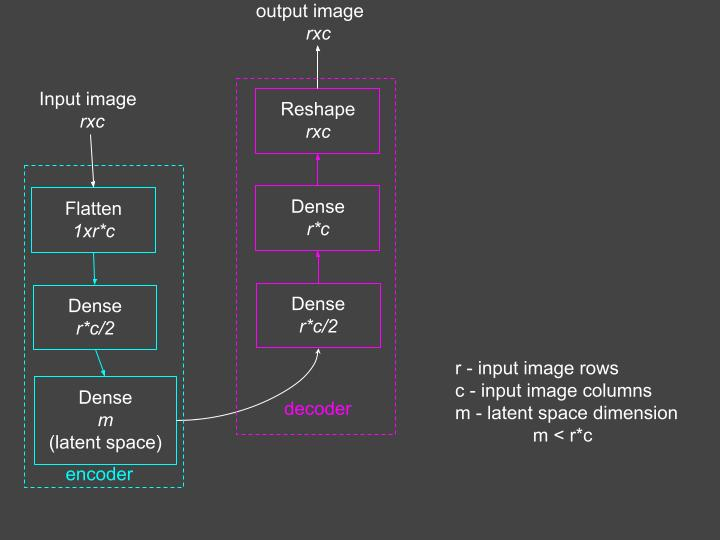Figure 1: The autoencoder model. Flatten and reshape layers reshape the received inputs without changing the values. Source: Mehreen Saeed

## The Import Section

Before starting the implementation, import the following libraries/modules in your code.

from tensorflow.keras import Sequential
from tensorflow.keras.models import Model
from tensorflow.keras.layers import Input, Dense
from tensorflow.keras.layers import Reshape, Flatten
# For dataset
from sklearn.datasets import fetch_lfw_people
from sklearn.model_selection import train_test_split
# For miscellaneous functions
from tensorflow.keras import utils
# For array functions
import numpy as np
# For plotting
import matplotlib.pyplot as plt
# For confusion matrix
from sklearn.metrics import confusion_matrix, ConfusionMatrixDisplay

## Load the ‘Labeled Faces in the Wild’ People Dataset

The scikit-learn library includes the Labeled Faces in the Wild (LFW) dataset, which consists of gray scale face images of different people. Because the dataset is imbalanced, we’ll load only the face images of people with at least 100 images included. We’ll also resize each image to half its dimensions to make the dataset more manageable.

Let’s load the dataset and display a few images along with some data statistics.

lfw_people = fetch_lfw_people(min_faces_per_person=100, resize=0.5)
# Get statistics of data
n_samples, r, c = lfw_people.images.shape
target_names = lfw_people.target_names
n_classes = target_names.shape

# Display first 8 images of dataset
total_cols = 8
fig, ax = plt.subplots(nrows=1, ncols=total_cols,
figsize=(18,4),
subplot_kw=dict(xticks=[], yticks=[]))

for j in range(total_cols):
ax[j].imshow(lfw_people.images[j, :, :])
plt.title('First 8 Dataset Images', y=-0.2, x=-4)
plt.show()

# Print statistics
print('STATISTICS')
print('Dataset has ', n_samples, 'sample images')
print('Each image is ', r, 'x', c)
print('Faces are of:\n', target_names)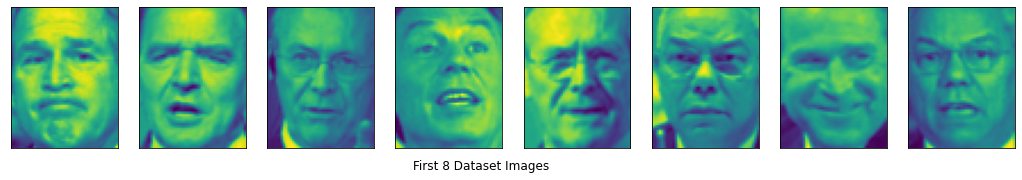STATISTICS
Dataset has 1140 sample images
Each image is 62 x 47
Faces are of:
['Colin Powell' 'Donald Rumsfeld' 'George W Bush' 'Gerhard Schroeder'
'Tony Blair']

### Prepare the Train and Test Data

The next step is to prepare the train and test data. The code below implements the following steps:

1. Use train_test_split() method to split the dataset into a 75% training and 25% test set.
2. Normalize each image’s pixel values to lie between 0 and 1. Because the grayscale images have pixel values between 0 and 255, we can divide each pixel value by 255 to normalize the entire image.
3. Use the to_categorical() method to convert each target value (range [0, 5]) to a five dimensional binary categorical vector.
4. Print the training and test data statistics.
X = lfw_people.images
y = lfw_people.target
# Create train and test sets
train_X, test_X, train_Y, test_Y = train_test_split(
X, y, test_size=0.25, random_state=0)
# Normalize each image
train_X = train_X/255
test_X = test_X/255
# Create 5 dimensional binary indicator vectors
train_Y_categorical = utils.to_categorical(train_Y)
test_Y_categorical = utils.to_categorical(test_Y)
# Print statistics
print("Training data shape: ", train_X.shape)
print("Training categorical labels shape: ", train_Y_categorical.shape)
print("Test data shape: ", test_X.shape)
print("Test categorical labels shape: ", test_Y_categorical.shape)
Training data shape: (855, 62, 47)
Training categorical labels shape: (855, 5)
Test data shape: (285, 62, 47)
Test categorical labels shape: (285, 5)

## Create the Autoencoder Model

The code below shows how you can use the Flatten, Dense and Reshape layers to create the autoencoder model shown in Figure 2. The value of the latent_dimension is set at 420. You can experiment with different values of this variable.

Because we want the input images to match the output images, we optimize with respect to the mean square error (mse). This can be specified as a parameter to the compile() method.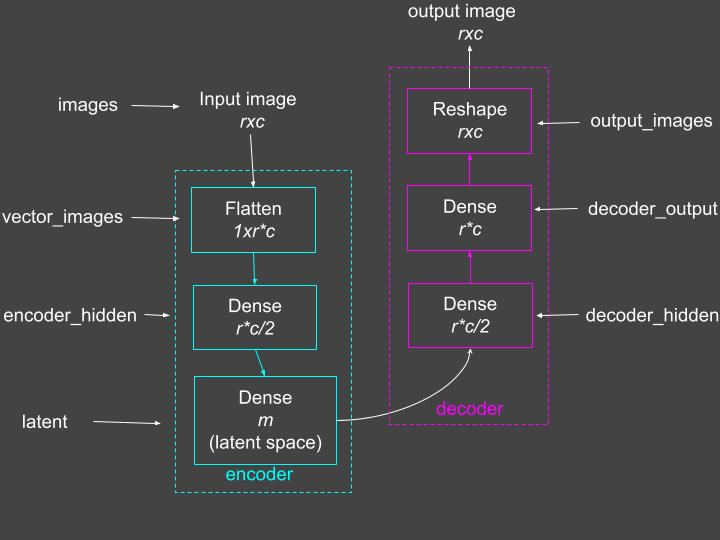Figure 2: The autoencoder model with layer names used in the code. Source: Mehreen Saeed

latent_dimension = 420
input_shape = (train_X.shape, train_X.shape)
n_inputs = input_shape*input_shape
images = Input(shape=(input_shape, input_shape, ))
vector_images = Flatten()(images)
# encoder
encoder_hidden = Dense(n_inputs/2, activation='relu')(vector_images)
latent = Dense(latent_dimension, activation='relu')(encoder_hidden)
# define decoder
decoder_hidden = Dense(n_inputs/2, activation='relu')(latent)
# output dense layer
decoder_output = Dense(n_inputs, activation='linear')(decoder_hidden)
output_images = Reshape((input_shape, input_shape, ))(decoder_output)
# define autoencoder model
autoencoder = Model(inputs=images, outputs=output_images)
# compile autoencoder model

Now let’s look at the summary of the autoencoder model we just built.

autoencoder.summary()
Model: "model"
_________________________________________________________________
Layer (type) Output Shape Param #
=================================================================
input_1 (InputLayer) [(None, 62, 47)] 0
_________________________________________________________________
flatten (Flatten) (None, 2914) 0
_________________________________________________________________
dense (Dense) (None, 1457) 4247155
_________________________________________________________________
dense_1 (Dense) (None, 420) 612360
_________________________________________________________________
dense_2 (Dense) (None, 1457) 613397
_________________________________________________________________
dense_3 (Dense) (None, 2914) 4248612
_________________________________________________________________
reshape (Reshape) (None, 62, 47) 0
=================================================================
Total params: 9,721,524
Trainable params: 9,721,524
Non-trainable params: 0
_________________________________________________________________

## Training the Autoencoder

Now we are ready to train the autoencoder model. The fit() method below trains the model and returns a history object with details of the entire training process.

# fit the autoencoder model to reconstruct input
history = autoencoder.fit(train_X, train_X, epochs=100, validation_split=0.33, verbose=0)

We can visualize the entire learning process by using the values stored in the dictionary object of history. The following code prints the keys of the history.history dictionary object and plots the training and validation loss for each epoch. As expected, the value of the loss function for the training set is lower than the loss value for the validation set.

print('Keys of history.history: ', history.history.keys())

fig = plt.figure(figsize=(15,4))
plt.plot(history.history['loss'])
plt.plot(history.history['val_loss'])
plt.legend(['Training loss', 'Validation loss'])
plt.title('Training and Validation Loss')
plt.xlabel('Epoch Number')
plt.ylabel('Loss')
plt.show()
Keys of history.history: dict_keys(['loss', 'val_loss'])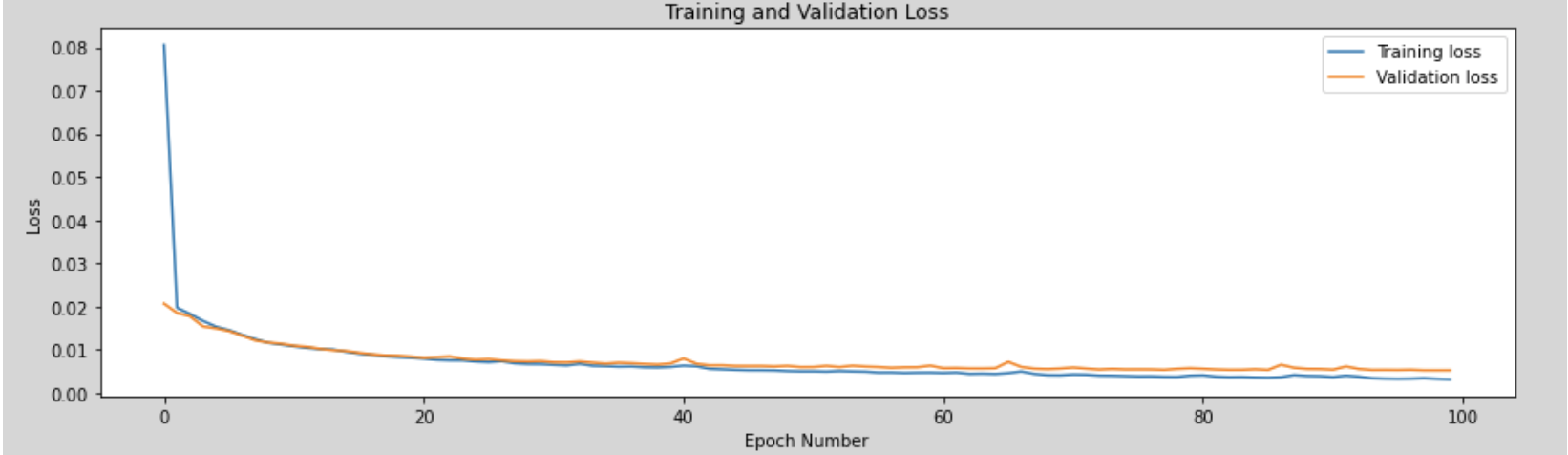## Reconstructing the Input Images

After training the autoencoder, we can look at what the reconstructed input images look like. The predict() method returns the output of the autoencoder for the inputs specified as a parameter. The code below displays the first eight images of the test set in the first row and their corresponding reconstructions in the second row.

# Reconstruction
reconstructed_test = autoencoder.predict(test_X)
# Display the inputs and reconstructions
total_cols = 8
fig, ax = plt.subplots(nrows=2, ncols=total_cols,
figsize=(18,4),
subplot_kw=dict(xticks=[], yticks=[]))

for j in range(total_cols):
ax[0, j].imshow(test_X[j, :, :])
ax[1, j].imshow(reconstructed_test[j, :, :])
plt.title('Input Images (Top Row) and Their Reconstruction by the Autoencoder (Bottom Row)', y=-0.4, x=-5)
plt.show()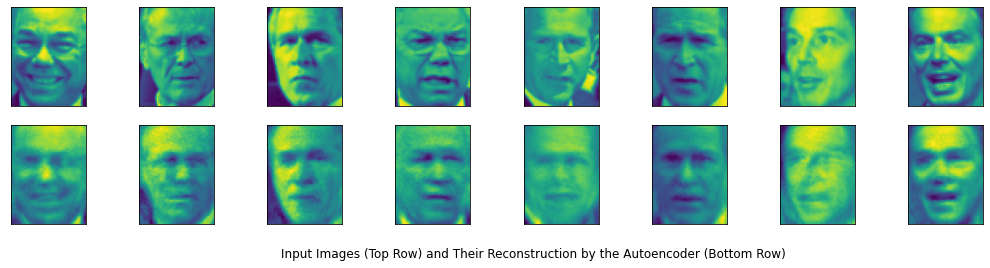The reconstructed images are quite interesting. We can see that they are an approximation of the original images and each closely replicates the facial expression of the input face. This makes them useful for augmenting a limited training set with more examples. You can generate as many images as needed by training the autoencoder multiple times. The reconstructed faces will vary with each run as the weights of the autoencoder are initialized randomly.

## Dissecting the Encoder

TensorFlow allows you to access the different layers of a model. You can easily retrieve the encoder block of the autoencoder by using the Model() method and instantiating it with the input images and output latent layer we created earlier. Let’s look at the summary of the encoder model.

# Encoder model
encoder_model = Model(inputs=images, outputs=latent)
encoder_model.summary()
Model: "model_1"
_________________________________________________________________
Layer (type) Output Shape Param #
=================================================================
input_1 (InputLayer) [(None, 62, 47)] 0
_________________________________________________________________
flatten (Flatten) (None, 2914) 0
_________________________________________________________________
dense (Dense) (None, 1457) 4247155
_________________________________________________________________
dense_1 (Dense) (None, 420) 612360
=================================================================
Total params: 4,859,515
Trainable params: 4,859,515
Non-trainable params: 0
_________________________________________________________________

The summary shows that we started out with an input of 2,914 (62x47) pixels and reduced it to only 420 outputs. These 420 outputs are an internal/latent space representation of the corresponding input image. The output of the encoder is hard for us to interpret. However, we can give it a try and visualize it by displaying it as an image. The code below arbitrarily reshapes the latent representation to a 20x21 image and renders it. The top row shows the input training image, and the bottom row shows the corresponding latent representation.

# Encoder output
encoder_output = encoder_model.predict(train_X)

latent_dim = encoder_output.shape
latent_shape = (20,21)
# Plot the first 8 images and their corresponding latent representation
total_cols = 8
fig, ax = plt.subplots(nrows=2, ncols=total_cols,
figsize=(18,4),
subplot_kw=dict(xticks=[], yticks=[]))

for j in range(total_cols):
train_image = train_X[j, :, :]
ax[0, j].imshow(train_image)
encoder_image = np.reshape(encoder_output[j, :], latent_shape)
ax[1, j].imshow(encoder_image)
plt.title('Input Images (Top Row) and Their Encoder Latent Representation (Bottom Row)', y=-0.4, x=-4)
plt.show()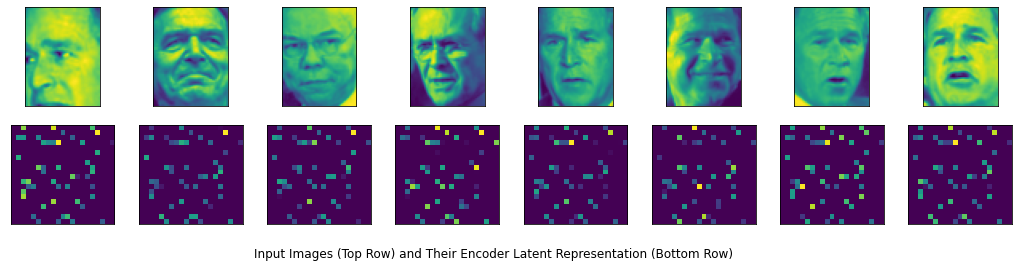## Using the Autoencoder for Supervised Learning

Strictly speaking, an autoencoder is not a supervised learning model, since it is trained with unlabeled images. However, we can use its latent representation to train a supervised learning model. The code below instantiates a classifier model by using all the layers of the autoencoder, from the vector_images layer up to the decoder_hidden layer. It then appends the model with a softmax layer that contains as many units as the number of classes/categories present in our dataset.

In the classifier model, we’ll set all the encoder layers to be nontrainable. This way the input image will be converted to its learned latent representation to be further processed by the classifier. The classifier will start training with the decoder weights tuned by the autoencoder and fine-tune them further to learn the classification of each latent representation. Figure 3 shows a block diagram of the classifier created from the autoencoder model. Now that we have a multiclass classification problem, we can use the ‘categorical_crossentropy’ as our loss function when compiling the model.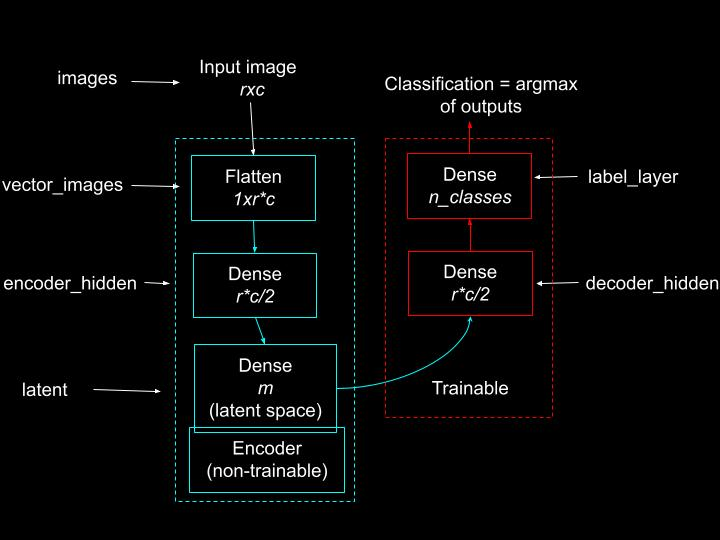Figure 3: The classifier model created from the encoder. Source: Mehreen Saeed

# Create classifier model
classifier = Sequential()
label_layer = Dense(n_classes, activation='softmax')(decoder_hidden)
classifier = Model(inputs=images, outputs=label_layer)

# Set the encoder layers to non-trainable
for layer in classifier.layers[:-2]:
layer.trainable=False

# Compile the model

Here is the summary of the classifier model. It has 620,687 trainable parameters associated with the last two layers. The rest of the weights up through the encoder layer are nontrainable.

classifier.summary()
Model: "model_2"
_________________________________________________________________
Layer (type) Output Shape Param #
=================================================================
input_1 (InputLayer) [(None, 62, 47)] 0
_________________________________________________________________
flatten (Flatten) (None, 2914) 0
_________________________________________________________________
dense (Dense) (None, 1457) 4247155
_________________________________________________________________
dense_1 (Dense) (None, 420) 612360
_________________________________________________________________
dense_2 (Dense) (None, 1457) 613397
_________________________________________________________________
dense_4 (Dense) (None, 5) 7290
=================================================================
Total params: 5,480,202
Trainable params: 620,687
Non-trainable params: 4,859,515
_________________________________________________________________

Let’s train the classifier using the fit() method.

history = classifier.fit(train_X, train_Y_categorical, epochs=150, validation_split=0.33, verbose=0)

### Evaluate the Classifier

The code below prints the classification accuracy on the training and test sets. Because this is a multiclass classification problem, it’s good to observe the confusion matrix. TensorFlow’s math module provides a routine for computing the confusion matrix, but we’ll use the method provided by the scikit-learn library. This library also includes a nice method for displaying the confusion matrix with fancy colors. Now you have reasonably good accuracy on the face classification task without doing any preprocessing, hyper-parameter tuning, and model selection.

train_loss, train_acc = classifier.evaluate(train_X, train_Y_categorical)
test_loss, test_acc = classifier.evaluate(test_X, test_Y_categorical)
print('Classification accuracy on training set: ', train_acc)
print('Classification accuracy on test set: ', test_acc)
27/27 [==============================] - 0s 6ms/step - loss: 0.2781 - accuracy: 0.9368
9/9 [==============================] - 0s 6ms/step - loss: 0.5743 - accuracy: 0.8596
Classification accuracy on training set: 0.9368420839309692
Classification accuracy on test set: 0.859649121761322
# Get the classifier outputs
test_predict = classifier.predict(test_X)
# Get the classification labels
test_predict_labels = np.argmax(test_predict, axis=1)
# Create and display the confusion matrix
test_confusion_matrix = confusion_matrix(test_Y, test_predict_labels)
cm = ConfusionMatrixDisplay(confusion_matrix=test_confusion_matrix,
display_labels=target_names)

cm.plot(xticks_rotation="vertical")
print('Correct classification: ', np.sum(np.diagonal(test_confusion_matrix)), '/', test_X.shape)

Correct classification: 245 / 285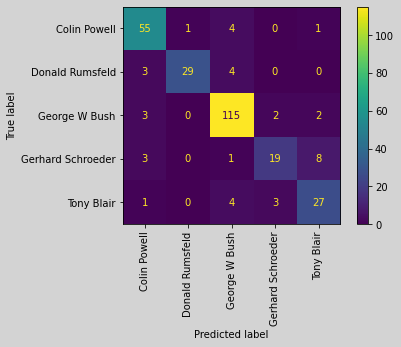## The Next Step

You just developed an autoencoder that can create an approximate representation of its inputs. You also used its latent representation to develop a classifier and applied it to a face recognition problem. While this may not be the best example to demonstrate the merits of the autoencoder as a supervised classifier, it should give you a fairly good idea of how to implement an autoencoder model and use its latent space representation for other tasks.

Now that you have a basic understanding of the autoencoder model, you can develop more advanced variants, such as a variational autoencoder or sparse autoencoder.

### You can run the entire code shown in this tutorial in Google Colab or download the Python notebook here.